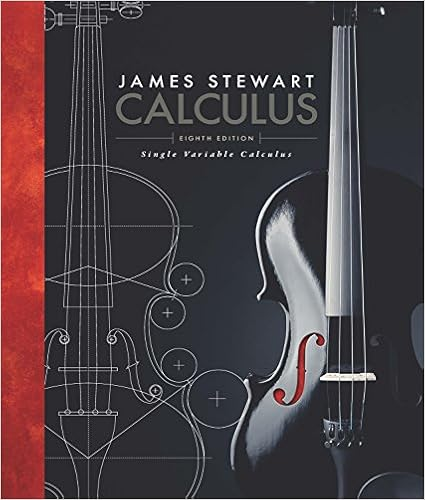# A 4365 b 4412 c 4459 d 5667 e 8176 7 if gx 025e 2x 6

• Notes
• MCQubed
• 3

This preview shows page 1 - 3 out of 3 pages.

##### We have textbook solutions for you!
The document you are viewing contains questions related to this textbook.The document you are viewing contains questions related to this textbook.
Chapter 2 / Exercise 5
Single Variable Calculus
StewartExpert Verified
car moving away from the radio tower 3 seconds after it passes through the intersection?A. 43.65B. 44.12C. 44.59D. 56.67E. 81.76
7. If g(x) = 0.25e2x-6+ (x – 2)5/2, then =)
g
##### We have textbook solutions for you!
The document you are viewing contains questions related to this textbook.The document you are viewing contains questions related to this textbook.
Chapter 2 / Exercise 5
Single Variable Calculus
StewartExpert Verified
8. If the base b of a triangle is increasing at a rate of 3 inches per minute while its height h is decreasing at a rate of 3 inches per minute, which of the following must be true about the area A of the triangle?
9. If f(x) = = (x)f then xx3,31x22
3x B.       3x
A.
2xxx2x D.
10. If f(x) = x3– x + 3 and c is the only real number such that f(c) = 0, then according to the Intermediate Value Theorem c must be in the interval
Free Response: Show all work.1. Differentiate: (a) (29100h(x)x1=+(b) 2y3x5x2=+-(c) r(x) = tan(3x2+ 1)2. A particle moves along the x-axis so that at time t its position is given by the equation s(t) = t3– 3t2+ 3 for t 0, where t is in seconds and s is in inches.(a) Assuming the particle is moving along the x-axis, during what intervals is the particle moving left? Right? Why?
•••